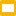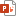## Spelling Bee in Scratch

Inspired by the NY Times Spelling Bee, I challenged myself to create a Scratch version of this game.
Rules: Words must be at least 4 letters long, and must contain the yellow center letter. Letters do not have to be adjacent to each other, and duplicating letter is allowed. I created the background hexagons with Google Drawings and Inkscape. In Scratch, I created a “dictionary” based on English word list from: http://www.mediafire.com/file/5cy3vcsag29ic43/Word_List.txt/file
I also created letter frequencies based the following sources:
https://www3.nd.edu/~busiforc/handouts/cryptography/letterfrequencies.html
https://en.wikipedia.org/wiki/Scrabble_letter_distributions
https://en.wikipedia.org/wiki/Letter_frequency

I got a great suggestion from Nirmala Sankaran, Co-Founder of HeyMath, to include the total possible words for a given board. This required iterating over the dictionary and checking if each word is valid for each board, given the game rules.

You can find the original code here: https://scratch.mit.edu/projects/603333011TANGRAMS by Avi MegiddoTANGRAMS by Avi Megiddo.pptx

• To play, you will need to edit this file, and you can make a copy by clicking
‘File’ –> ‘Make a copy’
• Click the ‘Shift’ key while rotating pieces to snap to common angles.
• Implementing Tangrams in Google Slides makes a lot of sense for online/blended learning. Solving and creating tangrams puzzles digitally builds multimedia literacy skills, visuospatial skills, design skills, and a deeper appreciaion for the affordances of Google Slides.
• This material is suitable for primary mathematics, PYP Design, and MYP Design
• Creating the Tangrams set from scratch in Google Drawings is a good high-ceiling activity, and a way to teach unit conversion, proportions, aspect ratio, and more.

## Base Ten Blocks in Google Slides

Counting, Adding, Multiplying, and Place Value 1 to 1000.
Math ages 5 – 9.Base Ten Blocks: Counting, Adding, Multiplying, and Place ValueBase Ten Blocks_ Counting, Adding, Multiplying, and Place Value for the numbers 1 to 1000.pptx

## Practice basic arithmetic, PEMDAS, build creativity

Subject: Math, Basic Operations, Mental Math
Grade Levels: 1st, 2nd, 3rd, 4th, 5th
Resource Type: Activities, Games, Google Apps
File Type: Google Slides™ (15 pages)
Practice basic arithmetic, PEMDAS, build creativity
To Use: Click on the gear icon and then click ‘Open editor’Make 24: Use only one number from each color group, and any operators +, -, x, /, and ( ) in Google Slides.

Practice basic arithmetic, PEMDAS, build creativity

Instructions:

→ Use exactly one number (1,2,3,4,5,6,7,8,9,10,11,12,13)
from each color group, and any operators +, -, x, /, and ( ),
to make an expression that equals 24.

→ Drag and drop the numbers and operators to the bottom bar

→ Go to the next slide and try to come up with another solution.

You can make your own version of the 24 game,
with different colors, fonts, sizes, and backgrounds.

Curriculum Standards:

CCSS
4.NBT.B.6
Find whole-number quotients and remainders with up to four-digit dividends and one-digit divisors, using strategies based on place value, the properties of operations, and/or the relationship between multiplication and division. Illustrate and explain the calculation by using equations, rectangular arrays, and/or area models.
CCSS
4.NBT.B.5
Multiply a whole number of up to four digits by a one-digit whole number, and multiply two two-digit numbers, using strategies based on place value and the properties of operations. Illustrate and explain the calculation by using equations, rectangular arrays, and/or area models.
CCSS
4.NBT.B.4
Fluently add and subtract multi-digit whole numbers using the standard algorithm.
CCSS
3.NBT.A.3
Multiply one-digit whole numbers by multiples of 10 in the range 10–90 (e.g., 9 × 80, 5 × 60) using strategies based on place value and properties of operations.
CCSS
3.NBT.A.2
Fluently add and subtract within 1000 using strategies and algorithms based on place value, properties of operations, and/or the relationship between addition and subtraction.
CCSS
2.NBT.B.9
Explain why addition and subtraction strategies work, using place value and the properties of operations.
CCSS
2.NBT.A.2
Count within 1000; skip-count by 5s, 10s, and 100s.
CCSS
1.NBT.C.5
Given a two-digit number, mentally find 10 more or 10 less than the number, without having to count; explain the reasoning used.
CCSS
1.NBT.C.4
Add within 100, including adding a two-digit number and a one-digit number, and adding a two-digit number and a multiple of 10, using concrete models or drawings and strategies based on place value, properties of operations, and/or the relationship between addition and subtraction; relate the strategy to a written method and explain the reasoning used. Understand that in adding two-digit numbers, one adds tens and tens, ones and ones; and sometimes it is necessary to compose a ten.

## Interactive Matchstick Math Puzzles in Google Sheets:MATCHSTICK Math PuzzlesMATCHSTICK Math Puzzles - Listed on TpT - Do Not Delete.pptx

## Design Thinking Challenge: Design a font for the numbers 0 through 9 with Google SheetsDesign Thinking Challenge: Make your own font for the numbers 0 through 9 - Listed on TpT - Do Not Delete

Subject: Arithmetic, Measurement, Mental Math
Grade Levels: PreK, Kindergarten, 1st, 2nd
Ages: 4-8 years old
Resource Type: Fun Stuff, Multimedia, Task Cards
File Type: Google Slides™ (28 pages)Number (Cuisenaire) Rods in Google Slides

“Cuisenaire rods used to illustrate the factors of ten
Cuisenaire rods are mathematics learning aids for students that provide an interactive, hands-on way to explore mathematics and learn mathematical concepts, such as the four basic arithmetical operations, working with fractions and finding divisors. In the early 1950s, Caleb Gattegno popularised this set of colored number rods created by the Belgian primary school teacher Georges Cuisenaire (1891–1975), who called the rods réglettes.
According to Gattegno, “Georges Cuisenaire showed in the early 1950s that students who had been taught traditionally and were rated ‘weak’, took huge strides when they shifted to using the material. They became ‘very good’ at traditional arithmetic when they were allowed to manipulate the rods.””

Simultaneously learn how to move, and rotate shapes, copy and paste between slides and import images.

Curriculum Standards:
CCSS
3.G.A.2
Partition shapes into parts with equal areas. Express the area of each part as a unit fraction of the whole. For example, partition a shape into 4 parts with equal area, and describe the area of each part as 1/4 of the area of the shape.
CCSS
2.G.A.2
Partition a rectangle into rows and columns of same-size squares and count to find the total number of them.
CCSS
1.G.A.2
Compose two-dimensional shapes (rectangles, squares, trapezoids, triangles, half-circles, and quarter-circles) or three-dimensional shapes (cubes, right rectangular prisms, right circular cones, and right circular cylinders) to create a composite shape, and compose new shapes from the composite shape.
CCSS
K.G.B.6
Compose simple shapes to form larger shapes. For example, “Can you join these two triangles with full sides touching to make a rectangle?”
CCSS
K.CC.C.6
Identify whether the number of objects in one group is greater than, less than, or equal to the number of objects in another group, e.g., by using matching and counting strategies.